# How much mesh refinement should I do?

A linear statics model calculates a maximum equivalent stress, which is compared with a yield strength.

An initial mesh has a certain element size around the point of maximum stress.
A second mesh with smaller elements is solved and gives a new value of maximum stress.
A third mesh with even smaller elements gives a third value of maximum stress.

How do you decide when to stop refining?

One method is described in ASME V&V10.1 “Illustration on V&V for Computational Solid Mechanics” and is called Calculation Verification, one small part of the entire Verification and Validation process.

Below is an example using a tetrahedral mesh with a Sizing Mesh Control on a Sphere of Influence.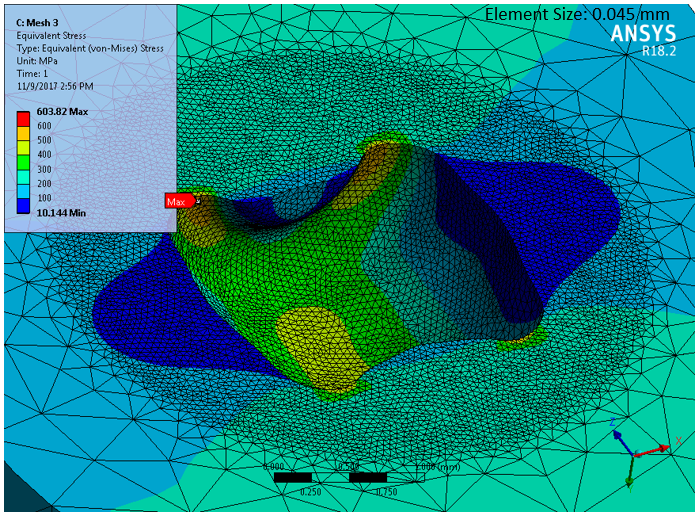Maximum Stress from six mesh sizes were plotted. The red line is a best fit line through the three smallest element sizes. This line, or the calculation in Section 7.2 of V&V10.1, can be used to extrapolate the maximum stress to a zero-size element. This is the estimate for the exact maximum stress, but is only valid when the results are being calculated in the asymptotic convergence regime. The line estimates the maximum stress is 607 MPa.  See this discussion for an alternative mesh control using inflation.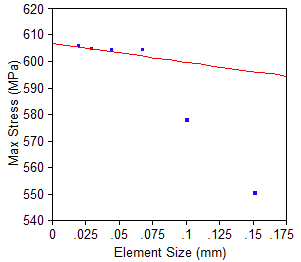The three largest element sizes are clearly trending toward a very different zero-size element value and should not be used since those results are not in the asymptotic convergence regime. V&V10.1 has more information on how to determine if you are in that regime.

In this example, I used a factor of 1.5 to change each successive element size. V&V10.1 recommends the factor be > 1.3.

«1

In your article and in the V&V10.1 it mentions about "asymptotic convergence regime", how can we know our results are in this region?

The explanation in the standard is bit confusing (V&V10.1). Which compares a theoretical and a calculated value for variable"p".

• Great question Rashi, and one that I had also pondered after finding the illustration and the standard to be lacking in clarity on that point.

Here is a tutorial that someone at NASA put together for CFD models. It has a paragraph on Asymptotic Range of Convergence.

• edited August 2018

Hi Peter,

for this example, is recommend use 0.1 or 0.15 element size?

• Hi Mekafime,

The element size is not an absolute value, and it would depend on the length units anyway.

The idea is that you let ANSYS mesh with an edge size that makes a reasonable looking mesh to get your first point on the plot.

Say in your case, the element edge length was 12 mm.  The rule is to divide by 1.5 to get the next point on the plot. So the series of solutions for the mesh size for elements around the peak stress would be: 12, 8, 5.3, 3.5, 2.4, 1.6 mm

You solve for each of those element sizes and plot the corresponding Maximum Stress.  Once you start to get a set of three or four points that are trending toward a straight line, you can stop cutting the element size.

Regards,

Peter

• edited August 2018

Hi Peter,

the size that you state is :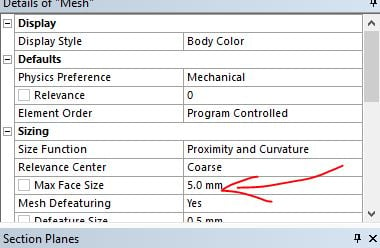and the stress value is the max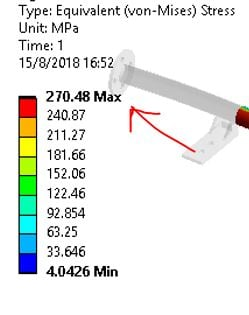Many values in size of mesh and stresses and with this I have the mesh convergence study?

• Hi mekafime,

Yes, that may work if you are on an unlimited Research license, since you are making a global mesh size change, you will get smaller elements everywhere, even away from the peak stress where the smaller elements are not needed.

If you are on a Student license, you might find that you exceed the allowable node count of 32,000 nodes before you have enough points on your mesh convergence plot when using the above mesh refinement approach.  A more efficient method is to apply localized sizing around the peak stress. Create a Coordinate System near the location of peak stress. Create a Sizing control on the bodies and set the size using a Sphere of Interest with an appropriate radius so that the smaller elements are inside that sphere, while outside that sphere the global mesh size is used.

Sometimes a model includes a stress singularity, which means that the stress keeps increasing as the element size decreases. Adding a blend to a sharp interior corner of the geometry in CAD is the usual mitigation to eliminate the stress singularity.

Regards,

Peter

• edited August 2018

Enough to deduce that your solution is mesh independent and you can then switch on to a Richardson extrapolation. Here some ideas from the NASA:

• Hi Peter,

I just found a problem like the one you mentioned about the stress singularity as the size of the elements is reduced. How I can add a blend to a Sharp interior corner ? Have you a video about this?

Thanks!

• How you add a blend depends on the Geometry editor you use. Is it DesignModeler, SpaceClaim or another CAD system?

• Hi Peter

DesignModeler and SolidWorks.

• In SolidWorks, you use the Fillet tool.

• Hi Peter,

In this model I have a fillet in a side because is a tube.I use sphere of influence with mesh size 1.3 factor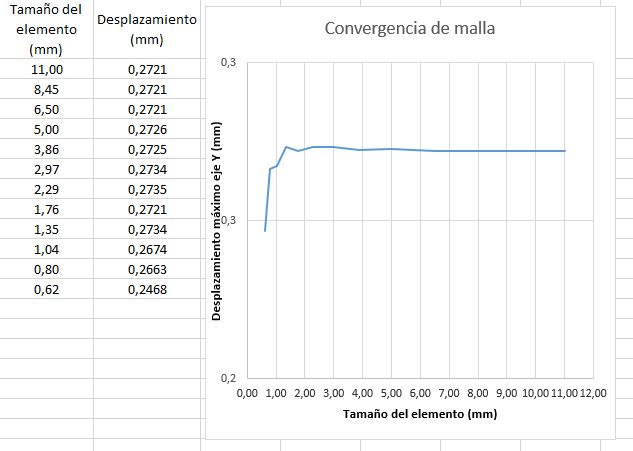I can use 10 mm for my model?

Thanks.

• What is the wall thickness?   What displacement are you measuring?

I suggest the sphere of influence include the fillet around both tubes instead of just the center portion.

• Hi Peter

Wall thickness = 5.92 mm

Displacement in Y (vertical)  from node in the middle of the tube, I'm refining the mesh of the horizontal tube.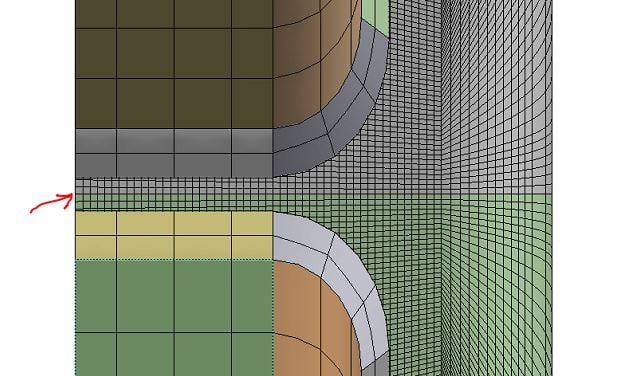Do you indicate that the sphere should be like this?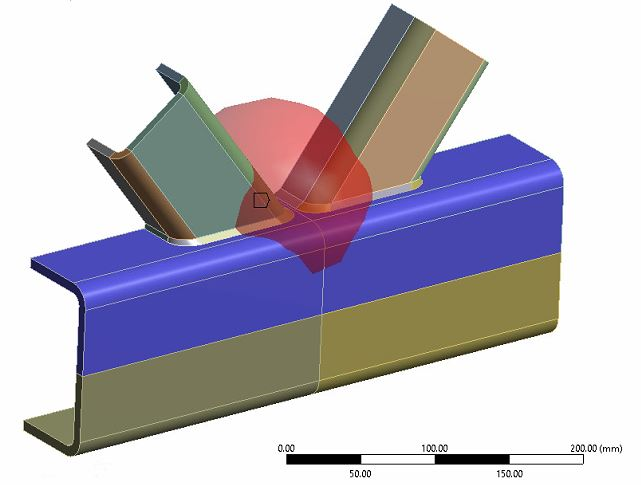Thanks

• I suggest you forget about the Sphere of Influence and just use the Mesh sizing control that is applied to the two tubes and the fillet. You can try size 10, 6.6, 4.4, 3.0, 2.0, 1.3 mm and plot that data.  What would be interesting is to see the effect on the results at a fixed element size, say 3 mm, of changing the Formulation of the contact.  MPC is probably the best.

I'm concerned that you have bonded contact in the region of high plasticity.  I refer you back to the post that I made before that I don't like contact used in regions of high plasticity.  This image represents an alternative way to make the connection. The bonded contact is far from the region of high plasticity which is occurring in a contiguous solid.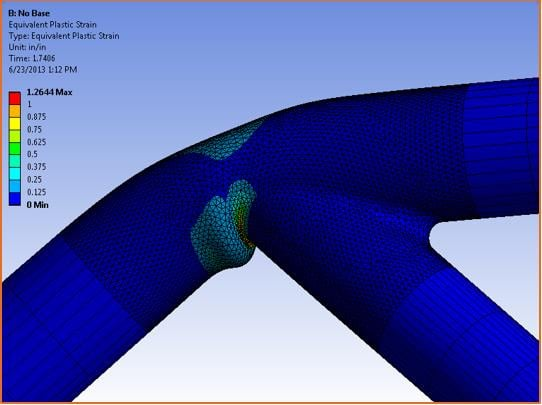• Hi Peter,

I have this assembly of 3 pieces (image) and I need only the central part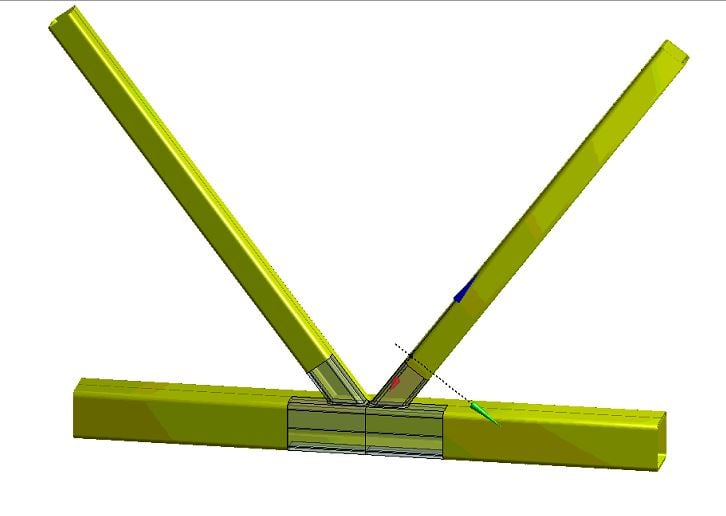I used cuts, trying to improve the mesh, the shared topology only apply on horizontal tube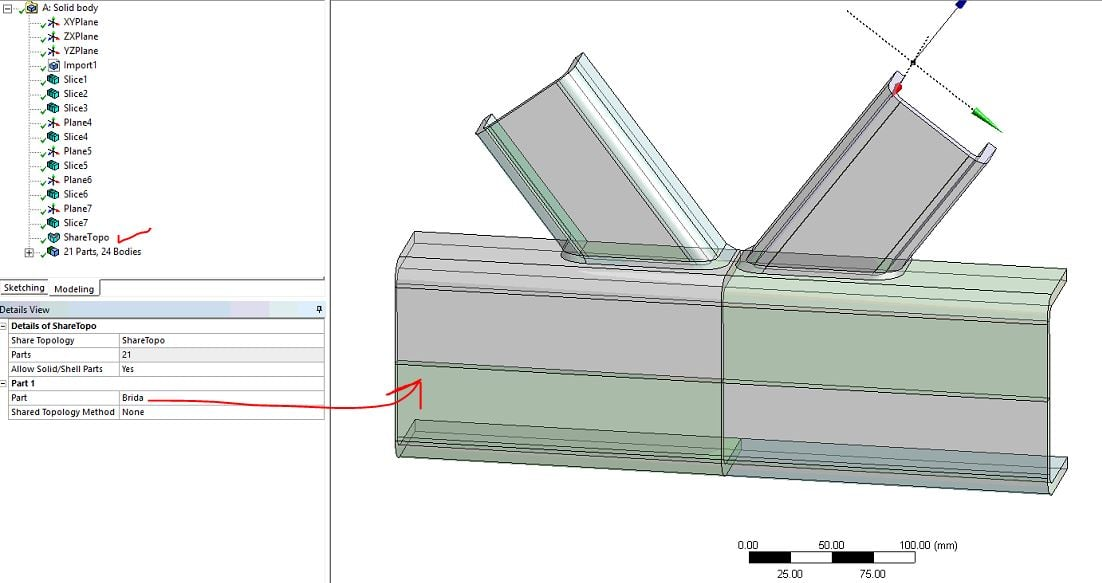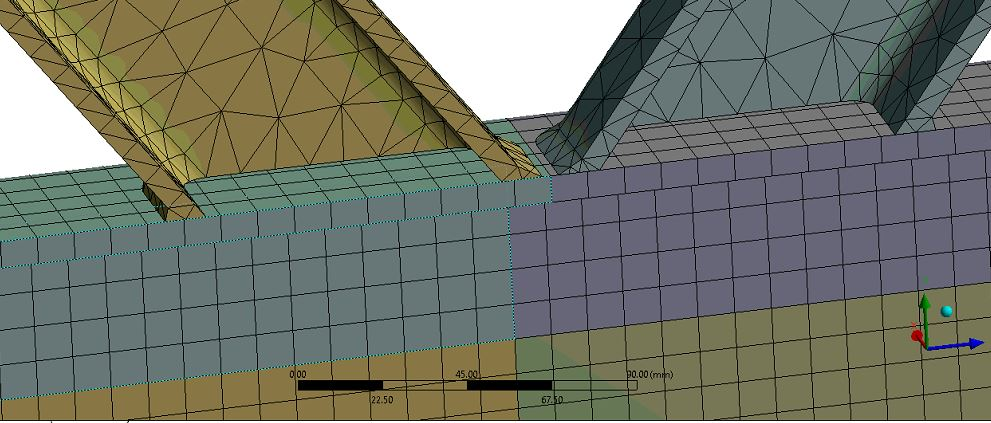What tool could I use in DesignModeler to merge the pieces?

Thanks.

• Boolean Unite

• Hi Peter,

I use Boolean , with some cuts because I need some points to take dates to graphics.

But when I do meshing is irregular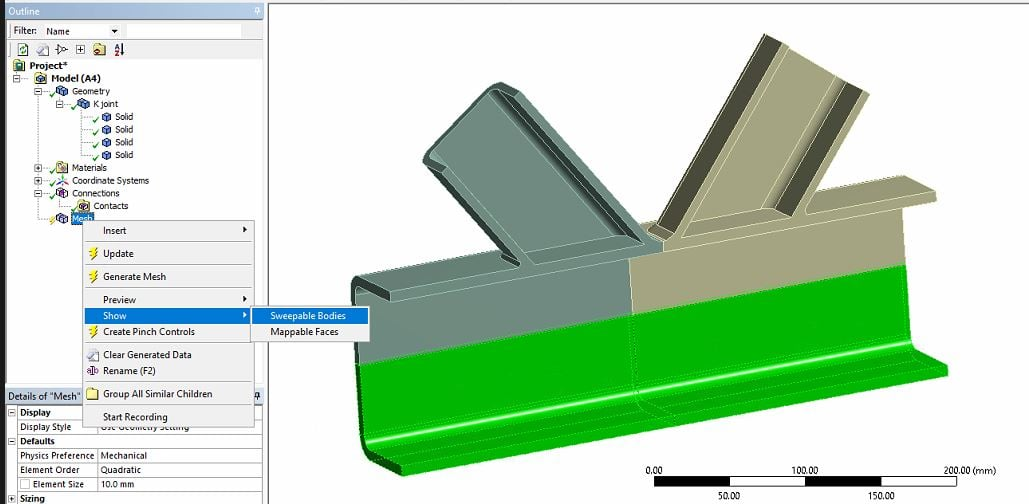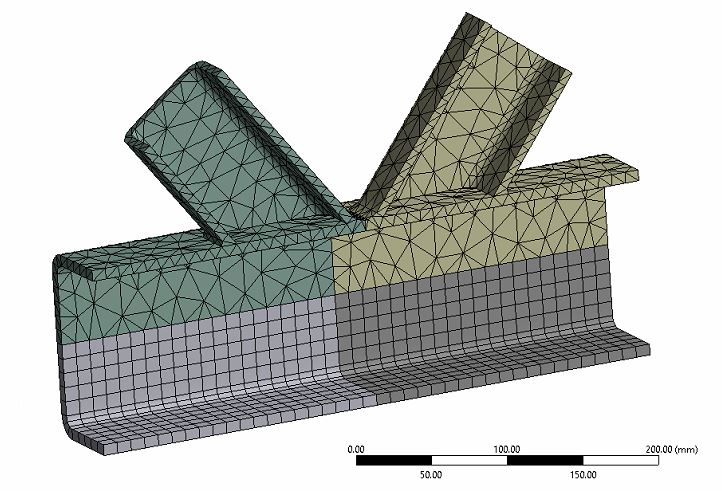I can´t use cuts because is the plasticity zone.

• I used hex dominant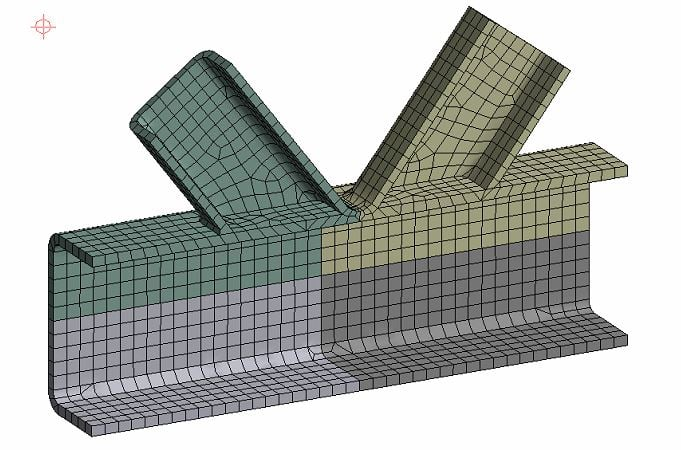• Great, now you can do the element size series for all those bodies meshed at 10 mm, 6.6 mm, 4.4 mm, 3.0 mm, 2.0 mm, 1.3 mm element size and plot the result data.  If you are using plasticity, Equivalent Total Strain is a good result to plot.